xHeat capacityOverview

Heat capacity or thermal capacity, is the measurable
Measurement
Measurement is the process or the result of determining the ratio of a physical quantity, such as a length, time, temperature etc., to a unit of measurement, such as the metre, second or degree Celsius...

physical quantity
Physical quantity
A physical quantity is a physical property of a phenomenon, body, or substance, that can be quantified by measurement.-Definition of a physical quantity:Formally, the International Vocabulary of Metrology, 3rd edition defines quantity as:...

that characterizes the amount of heat
Heat
In physics and thermodynamics, heat is energy transferred from one body, region, or thermodynamic system to another due to thermal contact or thermal radiation when the systems are at different temperatures. It is often described as one of the fundamental processes of energy transfer between...

required to change a substance's temperature
Temperature
Temperature is a physical property of matter that quantitatively expresses the common notions of hot and cold. Objects of low temperature are cold, while various degrees of higher temperatures are referred to as warm or hot...

by a given amount. In the International System of Units
International System of Units
The International System of Units is the modern form of the metric system and is generally a system of units of measurement devised around seven base units and the convenience of the number ten. The older metric system included several groups of units...

(SI), heat capacity is expressed in units of joule(s) (J)
Joule
The joule ; symbol J) is a derived unit of energy or work in the International System of Units. It is equal to the energy expended in applying a force of one newton through a distance of one metre , or in passing an electric current of one ampere through a resistance of one ohm for one second...

per kelvin (K)
Kelvin
The kelvin is a unit of measurement for temperature. It is one of the seven base units in the International System of Units and is assigned the unit symbol K. The Kelvin scale is an absolute, thermodynamic temperature scale using as its null point absolute zero, the temperature at which all...

.

Derived quantities that specify heat capacity as an intensive property, independent of the size of a sample, are the molar heat capacity, which is the heat capacity per mole
Mole (unit)
The mole is a unit of measurement used in chemistry to express amounts of a chemical substance, defined as an amount of a substance that contains as many elementary entities as there are atoms in 12 grams of pure carbon-12 , the isotope of carbon with atomic weight 12. This corresponds to a value...

of a pure substance, and the specific heat capacity, often simply called specific heat, which is the heat capacity per unit mass of a material.

Temperature
Temperature
Temperature is a physical property of matter that quantitatively expresses the common notions of hot and cold. Objects of low temperature are cold, while various degrees of higher temperatures are referred to as warm or hot...

reflects the average kinetic energy
Kinetic energy
The kinetic energy of an object is the energy which it possesses due to its motion.It is defined as the work needed to accelerate a body of a given mass from rest to its stated velocity. Having gained this energy during its acceleration, the body maintains this kinetic energy unless its speed changes...

of particles in matter.Unanswered QuestionsSpecific heat of plastic is 50 times larger than the specific heat of lead and ten times larger than the specific heat of stone. equal masses of lead,...Is heat retention the same as heat capacity??What are the factors effecting specific heat capacity,Hi. For calculating heat capacity (in Watts) Ph=q*p*cpdeltat where Ph is heat capacity in watts, q is the volume flow rate in cubic meters ...Encyclopedia
Heat capacity or thermal capacity, is the measurable
Measurement
Measurement is the process or the result of determining the ratio of a physical quantity, such as a length, time, temperature etc., to a unit of measurement, such as the metre, second or degree Celsius...

physical quantity
Physical quantity
A physical quantity is a physical property of a phenomenon, body, or substance, that can be quantified by measurement.-Definition of a physical quantity:Formally, the International Vocabulary of Metrology, 3rd edition defines quantity as:...

that characterizes the amount of heat
Heat
In physics and thermodynamics, heat is energy transferred from one body, region, or thermodynamic system to another due to thermal contact or thermal radiation when the systems are at different temperatures. It is often described as one of the fundamental processes of energy transfer between...

required to change a substance's temperature
Temperature
Temperature is a physical property of matter that quantitatively expresses the common notions of hot and cold. Objects of low temperature are cold, while various degrees of higher temperatures are referred to as warm or hot...

by a given amount. In the International System of Units
International System of Units
The International System of Units is the modern form of the metric system and is generally a system of units of measurement devised around seven base units and the convenience of the number ten. The older metric system included several groups of units...

(SI), heat capacity is expressed in units of joule(s) (J)
Joule
The joule ; symbol J) is a derived unit of energy or work in the International System of Units. It is equal to the energy expended in applying a force of one newton through a distance of one metre , or in passing an electric current of one ampere through a resistance of one ohm for one second...

per kelvin (K)
Kelvin
The kelvin is a unit of measurement for temperature. It is one of the seven base units in the International System of Units and is assigned the unit symbol K. The Kelvin scale is an absolute, thermodynamic temperature scale using as its null point absolute zero, the temperature at which all...

.

Derived quantities that specify heat capacity as an intensive property, independent of the size of a sample, are the molar heat capacity, which is the heat capacity per mole
Mole (unit)
The mole is a unit of measurement used in chemistry to express amounts of a chemical substance, defined as an amount of a substance that contains as many elementary entities as there are atoms in 12 grams of pure carbon-12 , the isotope of carbon with atomic weight 12. This corresponds to a value...

of a pure substance, and the specific heat capacity, often simply called specific heat, which is the heat capacity per unit mass of a material.

Temperature
Temperature
Temperature is a physical property of matter that quantitatively expresses the common notions of hot and cold. Objects of low temperature are cold, while various degrees of higher temperatures are referred to as warm or hot...

reflects the average kinetic energy
Kinetic energy
The kinetic energy of an object is the energy which it possesses due to its motion.It is defined as the work needed to accelerate a body of a given mass from rest to its stated velocity. Having gained this energy during its acceleration, the body maintains this kinetic energy unless its speed changes...

of particles in matter. Heat is the transfer of thermal energy; it flows from regions of high temperature to regions of low temperature. Thermal energy is stored as kinetic energy
Kinetic energy
The kinetic energy of an object is the energy which it possesses due to its motion.It is defined as the work needed to accelerate a body of a given mass from rest to its stated velocity. Having gained this energy during its acceleration, the body maintains this kinetic energy unless its speed changes...

in the random modes of translation in monatomic substances, and translations and rotations of polyatomic molecules in gases. Additionally, some thermal energy may be stored as the potential energy
Potential energy
In physics, potential energy is the energy stored in a body or in a system due to its position in a force field or due to its configuration. The SI unit of measure for energy and work is the Joule...

associated with higher-energy modes of vibration, whenever they occur in interatomic bonds in any substance. Translation, rotation, and a combination of the two types of energy in vibration (kinetic and potential) represent the degrees of freedom
Degrees of freedom (physics and chemistry)
A degree of freedom is an independent physical parameter, often called a dimension, in the formal description of the state of a physical system...

of motion which classically contribute to the heat capacity of a thermodynamic system. On a microscopic scale, each particle in a system absorbs heat energy among the few degrees of freedom available to it, and this absorption contributes to a specific heat capacity which classically approaches a maximum per mole of particles that is set by the Dulong-Petit law. The limit is achieved by many kinds of solids at room temperature.

For quantum mechanical reasons, at any given temperature, some of these degrees of freedom may be unavailable, or only partially available, to store thermal energy. In such cases, the specific heat capacity will be a fraction of the maximum. As the temperature approaches absolute zero
Absolute zero
Absolute zero is the theoretical temperature at which entropy reaches its minimum value. The laws of thermodynamics state that absolute zero cannot be reached using only thermodynamic means....

, the specific heat capacity of a system also approaches zero, due to loss of available degrees of freedom. Quantum theory
Quantum mechanics
Quantum mechanics, also known as quantum physics or quantum theory, is a branch of physics providing a mathematical description of much of the dual particle-like and wave-like behavior and interactions of energy and matter. It departs from classical mechanics primarily at the atomic and subatomic...

can be used to quantitatively predict specific heat capacities in simple systems.

## Background

Before the development of modern thermodynamics, it was thought that heat was a fluid, the so-called caloric. Bodies were capable of holding a certain amount of this fluid, hence the term heat capacity, named and first investigated by Joseph Black
Joseph Black
Joseph Black FRSE FRCPE FPSG was a Scottish physician and chemist, known for his discoveries of latent heat, specific heat, and carbon dioxide. He was professor of Medicine at University of Glasgow . James Watt, who was appointed as philosophical instrument maker at the same university...

in the 1750s. Today one instead discusses the internal energy
Internal energy
In thermodynamics, the internal energy is the total energy contained by a thermodynamic system. It is the energy needed to create the system, but excludes the energy to displace the system's surroundings, any energy associated with a move as a whole, or due to external force fields. Internal...

of a system. This is made up of its microscopic kinetic and potential energy. Heat is no longer considered a fluid. Rather, it is a transfer of disordered energy at the microscopic level. Nevertheless, at least in English, the term "heat capacity" survives. Some other languages prefer the term thermal capacity, which is also sometimes used in English.

## Extensive and intensive quantities

An object's heat capacity (symbol C) is defined as the ratio of the amount of heat energy transferred to an object to the resulting increase in temperature of the object,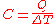In the International System of Units, heat capacity has the unit joules per kelvin.

Heat capacity is an extensive property, meaning it is a physical property that scales with the size of a physical system. A sample containing twice the amount of substance as another sample requires the transfer of twice the amount of heat (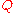) to achieve the same change in temperature (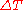).

For many experimental and theoretical purposes it is more convenient to report heat capacity as an intensive property - an intrinsic characteristic of a particular substance. This is most often accomplished by expressing the property in relation to a unit of mass
Mass
Mass can be defined as a quantitive measure of the resistance an object has to change in its velocity.In physics, mass commonly refers to any of the following three properties of matter, which have been shown experimentally to be equivalent:...

. In science and engineering, such properties are often prefixed with the term specific. International standards now recommend that specific heat capacity always refer to division by mass. The units for the specific heat capacity are.

In chemistry, heat capacity is often specified relative to one mole, the unit of amount of substance
Amount of substance
Amount of substance is a standards-defined quantity that measures the size of an ensemble of elementary entities, such as atoms, molecules, electrons, and other particles. It is sometimes referred to as chemical amount. The International System of Units defines the amount of substance to be...

, and is called the molar heat capacity. It has the unit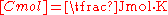.

For some considerations it is useful to specify the volume-specific heat capacity, commonly called volumetric heat capacity
Volumetric heat capacity
Volumetric heat capacity , also termed volume-specific heat capacity, describes the ability of a given volume of a substance to store internal energy while undergoing a given temperature change, but without undergoing a phase change...

, which is the heat capacity per unit volume and has SI units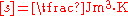. This is used almost exclusively for liquids and solids, since for gases it may be confused with specific heat capacity at constant volume.

## Metrology

The heat capacity of most systems is not a constant. Rather, it depends on the state variables of the thermodynamic system under study. In particular it is dependent on temperature itself, as well as on the pressure and the volume of the system.

Different measurements of heat capacity can therefore be performed, most commonly at constant pressure
Pressure
Pressure is the force per unit area applied in a direction perpendicular to the surface of an object. Gauge pressure is the pressure relative to the local atmospheric or ambient pressure.- Definition :...

and constant volume. The values thus measured are usually subscripted (by p and V, respectively) to indicate the definition. Gas
Gas
Gas is one of the three classical states of matter . Near absolute zero, a substance exists as a solid. As heat is added to this substance it melts into a liquid at its melting point , boils into a gas at its boiling point, and if heated high enough would enter a plasma state in which the electrons...

es and liquid
Liquid
Liquid is one of the three classical states of matter . Like a gas, a liquid is able to flow and take the shape of a container. Some liquids resist compression, while others can be compressed. Unlike a gas, a liquid does not disperse to fill every space of a container, and maintains a fairly...

s are typically also measured at constant volume. Measurements under constant pressure produce larger values than those at constant volume because the constant pressure values also include heat energy that is used to do work
Work (thermodynamics)
In thermodynamics, work performed by a system is the energy transferred to another system that is measured by the external generalized mechanical constraints on the system. As such, thermodynamic work is a generalization of the concept of mechanical work in mechanics. Thermodynamic work encompasses...

to expand the substance against the constant pressure as its temperature increases. This difference is particularly notable in gases where values under constant pressure are typically 30% to 66.7% greater than those at constant volume.

The specific heat capacities of substances comprising molecules (as distinct from monatomic gases) are not fixed constants and vary somewhat depending on temperature. Accordingly, the temperature at which the measurement is made is usually also specified. Examples of two common ways to cite the specific heat of a substance are as follows:
• Water (liquid): cp = 4.1855 [J/(g·K)] (15 °C, 101.325 kPa)
• Water (liquid): CvH = 74.539 J/(mol·K) (25 °C)

For liquids and gases, it is important to know the pressure for which given heat-capacity data refer. Most published data are given for standard pressure. However, quite different standard conditions for temperature and pressure
Standard conditions for temperature and pressure
Standard condition for temperature and pressure are standard sets of conditions for experimental measurements established to allow comparisons to be made between different sets of data...

have been defined by different organizations. The International Union of Pure and Applied Chemistry
International Union of Pure and Applied Chemistry
The International Union of Pure and Applied Chemistry is an international federation of National Adhering Organizations that represents chemists in individual countries. It is a member of the International Council for Science . The international headquarters of IUPAC is located in Zürich,...

(IUPAC) changed its recommendation from one atmosphere
Atmosphere (unit)
The standard atmosphere is an international reference pressure defined as 101325 Pa and formerly used as unit of pressure. For practical purposes it has been replaced by the bar which is 105 Pa...

to the round value 100 kPa (≈750.062 Torr).IUPAC.org, Gold Book, Standard Pressure. Besides being a round number, this had a very practical effect: relatively few people live and work at precisely sea level; 100 kPa equates to the mean pressure at an altitude of about 112 metres (which is closer to the 194–metre, world–wide median altitude of human habitation).

### Calculation

The path integral Monte Carlo
Path integral Monte Carlo
Path integral Monte Carlo is a quantum Monte Carlo method in the path integral formulation of quantum mechanics.The equations often are applied assuming that quantum exchange does not matter...

method is a numerical approach for determining the values of heat capacity, based on quantum dynamical principles.

### Alternative units

An older unit of heat is the kilogram-calorie
Calorie
The calorie is a pre-SI metric unit of energy. It was first defined by Nicolas Clément in 1824 as a unit of heat, entering French and English dictionaries between 1841 and 1867. In most fields its use is archaic, having been replaced by the SI unit of energy, the joule...

(Cal), originally defined as the energy required to raise the temperature of one kilogram of water by one degree Celsius, typically from 15°C to 16°C. The specific heat capacity of water on this scale would therefore be exactly 1 Cal/(K·kg). However, due to the temperature-dependence of the specific heat, a large number of different definitions of the calorie came into being. Whilst once it was very prevalent, especially its smaller cgs variant the gram-calorie (cal), defined so that the specific heat of water would be 1 cal/(K·g), in most fields the use of the calorie is now archaic.

In the United States other units of measure for heat capacity may be quoted in disciplines such as construction
Construction
In the fields of architecture and civil engineering, construction is a process that consists of the building or assembling of infrastructure. Far from being a single activity, large scale construction is a feat of human multitasking...

, civil engineering
Civil engineering
Civil engineering is a professional engineering discipline that deals with the design, construction, and maintenance of the physical and naturally built environment, including works like roads, bridges, canals, dams, and buildings...

, and chemical engineering
Chemical engineering
Chemical engineering is the branch of engineering that deals with physical science , and life sciences with mathematics and economics, to the process of converting raw materials or chemicals into more useful or valuable forms...

. A still common system is the English Engineering Units
English Engineering Units
Some fields of engineering in the United States use a system of measurement of physical quantities known as the English Engineering Units. The system is based on English units of measure.-Definition:...

in which the mass reference is pound mass
Pound (mass)
The pound or pound-mass is a unit of mass used in the Imperial, United States customary and other systems of measurement...

and the temperature is specified in degrees Fahrenheit
Fahrenheit
Fahrenheit is the temperature scale proposed in 1724 by, and named after, the German physicist Daniel Gabriel Fahrenheit . Within this scale, the freezing of water into ice is defined at 32 degrees, while the boiling point of water is defined to be 212 degrees...

or Rankine. One (rare) unit of heat is the pound calorie (lb-cal), defined as the amount of heat required to raise the temperature of one pound of water by one degree Celsius. On this scale the specific heat of water would be 1 lb-cal/(K·lb). More common is the British thermal unit
British thermal unit
The British thermal unit is a traditional unit of energy equal to about 1055 joules. It is approximately the amount of energy needed to heat of water, which is exactly one tenth of a UK gallon or about 0.1198 US gallons, from 39°F to 40°F...

, the standard unit of heat in the U.S. construction industry. This is defined such that the specific heat of water is 1 BTU/(°F·lb).

### Thermodynamic relations and definition of heat capacity

The internal energy of a closed system changes either by adding or removing heat to the system, or by the system performing work or having work done on it. Written mathematically we haveFor work as a result of compression or expansion of the system volume we may write,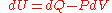If the process is performed at constant volume, then the second term of this relation vanishes and one readily obtains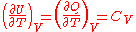This defines the heat capacity at constant volume,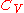.

Another useful quantity is the heat capacity at constant pressure,. We start with the enthalpy of the system given by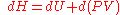which from our equation for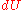simplifies to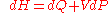, and therefore at constant pressure we have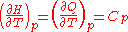.

### Relation between heat capacities

Measuring the heat capacity, sometimes referred to as specific heat, at constant volume can be prohibitively difficult for liquids and solids. That is, small temperature changes typically require large pressures to maintain a liquid or solid at constant volume implying the containing vessel must be nearly rigid or at least very strong (see coefficient of thermal expansion and compressibility). Instead it is easier to measure the heat capacity at constant pressure (allowing the material to expand or contract freely) and solve for the heat capacity at constant volume using mathematical relationships derived from the basic thermodynamic laws. Starting from the fundamental Thermodynamic Relation one can showwhere the partial derivatives are taken at constant volume and constant number of particles, and constant pressure and constant number of particles, respectively.

This can also be rewritten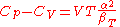whereis the coefficient of thermal expansion,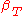is the isothermal compressibility.

The heat capacity ratio
Heat capacity ratio
The heat capacity ratio or adiabatic index or ratio of specific heats, is the ratio of the heat capacity at constant pressure to heat capacity at constant volume . It is sometimes also known as the isentropic expansion factor and is denoted by \gamma or \kappa . The latter symbol kappa is...

or adiabatic index is the ratio of the heat capacity at constant pressure to heat capacity at constant volume. It is sometimes also known as the isentropic expansion factor.

#### Ideal gas

For an ideal gas
Ideal gas
An ideal gas is a theoretical gas composed of a set of randomly-moving, non-interacting point particles. The ideal gas concept is useful because it obeys the ideal gas law, a simplified equation of state, and is amenable to analysis under statistical mechanics.At normal conditions such as...

, evaluating the partial derivatives above according to the equation of state
Equation of state
In physics and thermodynamics, an equation of state is a relation between state variables. More specifically, an equation of state is a thermodynamic equation describing the state of matter under a given set of physical conditions...the relation can be found to reduce to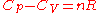where n is number of moles of gas in the thermodynamic system under consideration, and R is the universal gas constant.
Dividing through by n, this equation reduces simply to Mayer
Julius Robert von Mayer
Julius Robert von Mayer was a German physician and physicist and one of the founders of thermodynamics...

's relation,where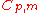and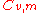are intensive property heat capacities expressed on a per mole basis at constant pressure and constant volume, respectively.

### Specific heat capacity

The specific heat capacity of a material on a per mass basis is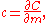which in the absence of phase transitions is equivalent towhereis the heat capacity of a body made of the material in question,is the mass of the body,is the volume of the body, andis the density of the material.

For gases, and also for other materials under high pressures, there is need to distinguish between different boundary conditions for the processes under consideration (since values differ significantly between different conditions). Typical processes for which a heat capacity may be defined include isobaric
Isobaric
Isobaric may refer to:*in thermodynamics, an isobaric process, i.e. one that is carried out at constant pressure;...

(constant pressure,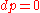) or isochoric
Isochoric process
An isochoric process, also called a constant-volume process, an isovolumetric process, or an isometric process, is a thermodynamic process during which the volume of the closed system undergoing such a process remains constant...

(constant volume,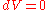) processes. The corresponding specific heat capacities are expressed as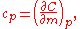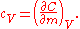From the results of the previous section, dividing through by the mass gives the relation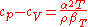A related parameter tois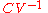, the volumetric heat capacity
Volumetric heat capacity
Volumetric heat capacity , also termed volume-specific heat capacity, describes the ability of a given volume of a substance to store internal energy while undergoing a given temperature change, but without undergoing a phase change...

. In engineering practice,for solids or liquids often signifies a volumetric heat capacity, rather than a constant-volume one. In such cases, the mass-specific heat capacity (specific heat) is often explicitly written with the subscript, as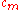. Of course, from the above relationships, for solids one writesFor pure homogeneous chemical compound
Chemical compound
A chemical compound is a pure chemical substance consisting of two or more different chemical elements that can be separated into simpler substances by chemical reactions. Chemical compounds have a unique and defined chemical structure; they consist of a fixed ratio of atoms that are held together...

s with established molecular or molar mass
Molecular mass
The molecular mass of a substance is the mass of one molecule of that substance, in unified atomic mass unit u...

or a molar quantity is established, heat capacity as an intensive property can be expressed on a per mole basis instead of a per mass basis by the following equations analogous to the per mass equations: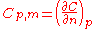= molar heat capacity at constant pressure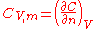= molar heat capacity at constant volume

where n = number of moles in the body or thermodynamic system
Thermodynamic system
A thermodynamic system is a precisely defined macroscopic region of the universe, often called a physical system, that is studied using the principles of thermodynamics....

. One may refer to such a per mole quantity as molar heat capacity to distinguish it from specific heat capacity on a per mass basis.

### Polytropic heat capacity

The polytropic heat capacity is calculated at processes if all the thermodynamic properties (pressure, volume, temperature) change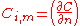= molar heat capacity at polytropic process

The most important polytropic processes run between the adiabatic and the isotherm functions, the polytropic index is between 1 and the adiabatic exponent (γ or κ)

### Dimensionless heat capacity

The dimensionless heat capacity of a material iswhere
C is the heat capacity of a body made of the material in question (J/K)
n is the amount of substance
Amount of substance
Amount of substance is a standards-defined quantity that measures the size of an ensemble of elementary entities, such as atoms, molecules, electrons, and other particles. It is sometimes referred to as chemical amount. The International System of Units defines the amount of substance to be...

in the body (mol
Mole (unit)
The mole is a unit of measurement used in chemistry to express amounts of a chemical substance, defined as an amount of a substance that contains as many elementary entities as there are atoms in 12 grams of pure carbon-12 , the isotope of carbon with atomic weight 12. This corresponds to a value...

)
R is the gas constant
Gas constant
The gas constant is a physical constant which is featured in many fundamental equations in the physical sciences, such as the ideal gas law and the Nernst equation. It is equivalent to the Boltzmann constant, but expressed in units of energy The gas constant (also known as the molar, universal,...

(J/(K·mol))
N is the number of molecules in the body. (dimensionless)
k is Boltzmann’s constant (J/(K·molecule))

In the ideal gas
Ideal gas
An ideal gas is a theoretical gas composed of a set of randomly-moving, non-interacting point particles. The ideal gas concept is useful because it obeys the ideal gas law, a simplified equation of state, and is amenable to analysis under statistical mechanics.At normal conditions such as...

article, dimensionless heat capacityis expressed as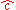, and is related there directly to half the number of degrees of freedom per particle. This holds true for quadratic degrees of freedom, a consequence of the equipartition theorem
Equipartition theorem
In classical statistical mechanics, the equipartition theorem is a general formula that relates the temperature of a system with its average energies. The equipartition theorem is also known as the law of equipartition, equipartition of energy, or simply equipartition...

.

More generally, the dimensionless heat capacity relates the logarithmic increase in temperature to the increase in the dimensionless entropy per particle, measured in nat
Nat (information)
A nat is a logarithmic unit of information or entropy, based on natural logarithms and powers of e, rather than the powers of 2 and base 2 logarithms which define the bit. The nat is the natural unit for information entropy...

s.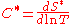Alternatively, using base 2 logarithms, C* relates the base-2 logarithmic increase in temperature to the increase in the dimensionless entropy measured in bit
Bit
A bit is the basic unit of information in computing and telecommunications; it is the amount of information stored by a digital device or other physical system that exists in one of two possible distinct states...

s.

### Heat capacity at absolute zero

From the definition of entropythe absolute entropy can be calculated by integrating from zero kelvins temperature to the final temperature Tf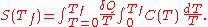The heat capacity must be zero at zero temperature in order for the above integral not to yield an infinite absolute entropy, which would violate the third law of thermodynamics
Third law of thermodynamics
The third law of thermodynamics is a statistical law of nature regarding entropy:For other materials, the residual entropy is not necessarily zero, although it is always zero for a perfect crystal in which there is only one possible ground state.-History:...

. One of the strengths of the Debye model
Debye model
In thermodynamics and solid state physics, the Debye model is a method developed by Peter Debye in 1912 for estimating the phonon contribution to the specific heat in a solid. It treats the vibrations of the atomic lattice as phonons in a box, in contrast to the Einstein model, which treats the...

is that (unlike the preceding Einstein model) it predicts the proper mathematical form of the approach of heat capacity toward zero, as absolute zero temperature is approached.

### Negative heat capacity (stars)

Most physical systems exhibit a positive heat capacity. However, even though it can seem paradoxical at first, there are some systems for which the heat capacity is negative. These include gravitating objects such as stars; and also sometimes some nano-scale
Nanoscopic scale
The nanoscopic scale usually refers to structures with a length scale applicable to nanotechnology, usually cited as 1-100 nanometers. The nanoscopic scale is a lower bound to the mesoscopic scale for most solids....

clusters of a few tens of atoms, close to a phase transition. A negative heat capacity can result in a negative temperature
Negative temperature
In physics, certain systems can achieve negative temperatures; that is, their thermodynamic temperature can be a negative quantity. Negative temperatures can be expressed as negative numbers on the kelvin scale....

.

According to the virial theorem, for a self-gravitating body like a star or an interstellar gas cloud, the average potential energy UPot and the average kinetic energy UKin are locked together in the relation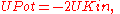The total energy U (= UPot + UKin) therefore obeys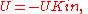If the system loses energy, for example by radiating energy away into space, the average kinetic energy and with it the average temperature actually increases. The system therefore can be said to have a negative heat capacity.

A more extreme version of this occurs with black hole
Black hole
A black hole is a region of spacetime from which nothing, not even light, can escape. The theory of general relativity predicts that a sufficiently compact mass will deform spacetime to form a black hole. Around a black hole there is a mathematically defined surface called an event horizon that...

s. According to black hole thermodynamics
Black hole thermodynamics
In physics, black hole thermodynamics is the area of study that seeks to reconcile the laws of thermodynamics with the existence of black hole event horizons...

, the more mass and energy a black hole absorbs, the colder it becomes. In contrast, if it is a net emitter of energy, through Hawking radiation
Hawking radiation
Hawking radiation is a thermal radiation with a black body spectrum predicted to be emitted by black holes due to quantum effects. It is named after the physicist Stephen Hawking, who provided a theoretical argument for its existence in 1974, and sometimes also after the physicist Jacob Bekenstein...

, it will become hotter and hotter until it boils away.

### Factors that affect specific heat capacity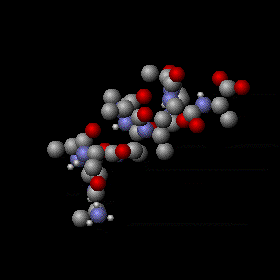For any given substance, the heat capacity of a body is directly proportional to the amount of substance it contains (measured in terms of mass or moles or volume). Doubling the amount of substance in a body doubles its heat capacity, etc.

However, when this effect has been corrected for, by dividing the heat capacity by the quantity of substance in a body, the resulting specific heat capacity is a function of the structure of the substance itself. In particular, it depends on the number of degrees of freedom
Degrees of freedom (physics and chemistry)
A degree of freedom is an independent physical parameter, often called a dimension, in the formal description of the state of a physical system...

that are available to the particles in the substance, each of which type of freedom allows substance particles to store thermal energy. The kinetic energy
Kinetic energy
The kinetic energy of an object is the energy which it possesses due to its motion.It is defined as the work needed to accelerate a body of a given mass from rest to its stated velocity. Having gained this energy during its acceleration, the body maintains this kinetic energy unless its speed changes...

of substance particles is the only one of the many possible degrees of freedom which manifests as temperature change, and thus the larger the number of degrees of freedom available to the particles of a substance other than kinetic energy, the larger will be the specific heat capacity for the substance.

In addition, quantum effects require that whenever energy be stored in any mechanism associated with a bound system which confers a degree of freedom, it must be stored in certain minimal-sized deposits (quanta) of energy, or else not stored at all. Such effects limit the full ability of some degrees of freedom to store energy when their lowest energy storage quantum amount is not easily supplied at the average energy of particles at a given temperature. In general, for this reason, specific heat capacities tend to fall at lower temperatures where the average thermal energy available to each particle degree of freedom is smaller, and thermal energy storage begins to be limited by these quantum effects. Due to this process, as temperature falls toward absolute zero, so also does heat capacity.

#### Degrees of freedom

Molecules are quite different from the monatomic gases like helium
Helium
Helium is the chemical element with atomic number 2 and an atomic weight of 4.002602, which is represented by the symbol He. It is a colorless, odorless, tasteless, non-toxic, inert, monatomic gas that heads the noble gas group in the periodic table...

and argon
Argon
Argon is a chemical element represented by the symbol Ar. Argon has atomic number 18 and is the third element in group 18 of the periodic table . Argon is the third most common gas in the Earth's atmosphere, at 0.93%, making it more common than carbon dioxide...

. With monatomic gases, thermal energy comprises only translational motions. Translational motions are ordinary, whole-body movements in 3D space
Three-dimensional space
Three-dimensional space is a geometric 3-parameters model of the physical universe in which we live. These three dimensions are commonly called length, width, and depth , although any three directions can be chosen, provided that they do not lie in the same plane.In physics and mathematics, a...

whereby particles move about and exchange energy in collisions—like rubber balls in a vigorously shaken container (see animation here). These simple movements in the three dimensions of space mean individual atoms have three translational degrees of freedom
Degrees of freedom (physics and chemistry)
A degree of freedom is an independent physical parameter, often called a dimension, in the formal description of the state of a physical system...

. A degree of freedom is any form of energy in which heat transferred into an object can be stored. This can be in translational kinetic energy, rotational kinetic energy, or other forms such as potential energy
Potential energy
In physics, potential energy is the energy stored in a body or in a system due to its position in a force field or due to its configuration. The SI unit of measure for energy and work is the Joule...

in vibrational modes. Only three translational degrees of freedom (corresponding to the three independent directions in space) are available for any individual atom, whether it is free, as a monatomic molecule, or bound into a polyatomic molecule.

As to rotation about an atom's axis (again, whether the atom is bound or free), its energy of rotation is proportional to the moment of inertia
Moment of inertia
In classical mechanics, moment of inertia, also called mass moment of inertia, rotational inertia, polar moment of inertia of mass, or the angular mass, is a measure of an object's resistance to changes to its rotation. It is the inertia of a rotating body with respect to its rotation...

for the atom, which is extremely small compared to moments of inertia of collections of atoms. This is because almost all of the mass of a single atom is concentrated in its nucleus, which has a radius too small to give a significant moment of inertia. In contrast, the spacing of quantum energy levels for a rotating object is inversely proportional to its moment of inertia, and so this spacing becomes very large for objects with very small moments of inertia. For these reasons, the contribution from rotation of atoms on their axes is essentially zero in monatomic gases, because the energy spacing of the associated quantum levels is too large for significant thermal energy to be stored in rotation of systems with such small moments of inertia. For similar reasons, axial rotation around bonds joining atoms in diatomic gases (or along the linear axis in a linear molecule of any length) can also be neglected as a possible "degree of freedom" as well, since such rotation is similar to rotation of monatomic atoms, and so occurs about an axis with a moment of inertia too small to be able to store significant heat energy.

In polyatomic molecules, other rotational modes may become active, due to the much higher moments of inertia about certain axes which do not coincide with the linear axis of a linear molecule. These modes take the place of some translational degrees of freedom for individual atoms, since the atoms are moving in 3-D space, as the molecule rotates. The narrowing of quantum mechanically determined energy spacing between rotational states results from situations where atoms are rotating around an axis that does not connect them, and thus form an assembly that has a large moment of inertia. This small difference between energy states allows the kinetic energy of this type of rotational motion to store heat energy at ambient temperatures. Furthermore (although usually at higher temperatures than are able to store heat in rotational motion) internal vibrational degrees of freedom also may become active (these are also a type of translation, as seen from the view of each atom). In summary, molecules are complex objects with a population of atoms that may move about within the molecule in a number of different ways (see animation at right), and each of these ways of moving is capable of storing energy if the temperature is sufficient.

The heat capacity of molecules (on a per-atom, or atom-molar, basis) does not exceed the heat capacity of monatomic gases, unless vibrational modes are brought into play. The reason for this is that vibrational modes allow energy to be stored as potential energy in intra-atomic bonds in a molecule, which are not available to atoms in monatomic gases. Up to about twice as much energy (on a per-atom basis) per unit of temperature increase can be stored in a solid as in a monatomic gas, by this mechanism of storing energy in the potentials of interatomic bonds. This gives many solids about twice the atom-molar heat capacity of monatomic gases, at the highest temperatures their structure can withstand.

However, quantum effects heavily affect the actual ratio at lower temperatures, especially in solids with light and tightly bound atoms (e.g., beryllium metal). Smaller polyatomic gases store intermediate amounts of energy, giving them a per-atom heat capacity that is between that of monatomic gases ( R per mole, where R is the ideal gas constant), and the maximum of fully excited warmer solids (3 R per mole). For gases, heat capacity never falls below the minimum of R per mole, since the kinetic energy of gas molecules is always available to store this much heat energy. However, for cryogenic temperatures in solids, heat capacity falls toward zero, as temperature approaches absolute zero.

#### Example of temperature-dependent specific heat capacity, in a diatomic gas

To illustrate the role of various degrees of freedom in storing heat, we may consider nitrogen
Nitrogen
Nitrogen is a chemical element that has the symbol N, atomic number of 7 and atomic mass 14.00674 u. Elemental nitrogen is a colorless, odorless, tasteless, and mostly inert diatomic gas at standard conditions, constituting 78.08% by volume of Earth's atmosphere...

, a diatomic
Diatomic
Diatomic molecules are molecules composed only of two atoms, of either the same or different chemical elements. The prefix di- means two in Greek. Common diatomic molecules are hydrogen , nitrogen , oxygen , and carbon monoxide . Seven elements exist in the diatomic state in the liquid and solid...

molecule that has five active degrees of freedom at room temperature: the three comprising translational motion plus two rotational degrees of freedom internally. Although the constant-volume molar heat capacity of nitrogen at this temperature is five-thirds that of monatomic gases, on a per-mole of atoms basis, it is five-sixths that of a monatomic gas. The reason for this is the loss of a degree of freedom due to the bond when it does not allow storage of thermal energy. Two separate nitrogen atoms would have a total of six degrees of freedom—the three translational degrees of freedom of each atom. When the atoms are bonded the molecule will still only have three translational degrees of freedom, as the two atoms in the molecule move as one. However, the molecule cannot be treated as a point object, and the moment of inertia has increased sufficiently about two axes to allow two rotational degrees of freedom to be active at room temperature to give five degrees of freedom. The moment of inertia about the third axis remains small, as this is the axis passing through the centres of the two atoms, and so is similar to the small moment of inertia for atoms of a monatomic gas. Thus, this degree of freedom does not act to store heat, and does not contribute to the heat capacity of nitrogen. The heat capacity per atom for nitrogen (5/2 per mole molecules = 5/4 per mole atoms) is therefore less than for a monatomic gas (3/2 per mole molecules or atoms), so long as the temperature remains low enough that no vibrational degrees of freedom are activated.

At higher temperatures, however, nitrogen gas gains two more degrees of internal freedom, as the molecule is excited into higher vibrational modes that store thermal energy. Now the bond is contributing heat capacity, and is contributing more than if the atoms were not bonded. With full thermal excitation of bond vibration, the heat capacity per volume, or per mole of gas molecules approaches seven-thirds that of monatomic gases. Significantly, this is seven-sixths of the monatomic gas value on a mole-of-atoms basis, so this is now a higher heat capacity per atom than the monatomic figure, because the vibrational mode enables for diatomic gases allows an extra degree of potential energy freedom per pair of atoms, which monatomic gases cannot possess. See thermodynamic temperature
Thermodynamic temperature
Thermodynamic temperature is the absolute measure of temperature and is one of the principal parameters of thermodynamics. Thermodynamic temperature is an "absolute" scale because it is the measure of the fundamental property underlying temperature: its null or zero point, absolute zero, is the...

for more information on translational motions, kinetic (heat) energy, and their relationship to temperature.

However, even at these large temperatures where gaseous nitrogen is able to store 7/6ths of the energy per atom of a monatomic gas (making it more efficient at storing energy on an atomic basis), it still only stores 7/12 ths of the maximal per-atom heat capacity of a solid, meaning it is not nearly as efficient at storing thermal energy on an atomic basis, as solid substances can be. This is typical of gases, and results because many of the potential bonds which might be storing potential energy in gaseous nitrogen (as opposed to solid nitrogen) are lacking, because only one of the spatial dimensions for each nitrogen atom offers a bond into which potential energy can be stored without increasing the kinetic energy of the atom. In general, solids are most efficient, on an atomic basis, at storing thermal energy (that is, they have the highest per-atom or per-mole-of-atoms heat capacity).
##### Per mole of molecules

When the specific heat capacity, c, of a material is measured (lowercase c means the unit quantity is in terms of mass), different values arise because different substances have different molar mass
Molar mass
Molar mass, symbol M, is a physical property of a given substance , namely its mass per amount of substance. The base SI unit for mass is the kilogram and that for amount of substance is the mole. Thus, the derived unit for molar mass is kg/mol...

es (essentially, the weight of the individual atoms or molecules). In solids, thermal energy arises due to the number of atoms that are vibrating. "Molar" heat capacity per mole of molecules, for both gases and solids, offer figures which are arbitrarily large, since molecules may be arbitrarily large. Such heat capacities are thus not intensive quantities for this reason, since the quantity of mass being considered can be increased without limit.
##### Per mole of atoms

Conversely, for molecular-based substances (which also absorb heat into their internal degrees of freedom), massive, complex molecules with high atomic count—like octane—can store a great deal of energy per mole and yet are quite unremarkable on a mass basis, or on a per-atom basis. This is because, in fully excited systems, heat is stored independently by each atom in a substance, not primarily by the bulk motion of molecules.

Thus, it is the heat capacity per-mole-of-atoms, not per-mole-of-molecules, which is the intensive quantity, and which comes closest to being a constant for all substances at high temperatures. This relationship was noticed empirically in 1819, and is called the Dulong-Petit law, after its two discoverers. Historically, the fact that specific heat capacities are approximately equal when corrected by the presumed weight of the atoms of solids, was an important piece of data in favor of the atomic theory of matter.

Because of the connection of heat capacity to the number of atoms, some care should be taken to specify a mole-of-molecules basis vs. a mole-of-atoms basis, when comparing specific heat capacities of molecular solids and gases. Ideal gases have the same numbers of molecules per volume, so increasing molecular complexity adds heat capacity on a per-volume and per-mole-of-molecules basis, but may lower or raise heat capacity on a per-atom basis, depending on whether the temperature is sufficient to store energy as atomic vibration.

In solids, the quantitative limit of heat capacity in general is about 3 R per mole of atoms, where R is the ideal gas constant. This 3 R value is about 24.9 J/mole.K. Six degrees of freedom (three kinetic and three potential) are available to each atom. Each of these six contributes R specific heat capacity per mole of atoms. This limit of 3 R per mole specific heat capacity is approached at room temperature for most solids, with significant departures at this temperature only for solids composed of the lightest atoms which are bound very strongly, such as beryllium
Beryllium
Beryllium is the chemical element with the symbol Be and atomic number 4. It is a divalent element which occurs naturally only in combination with other elements in minerals. Notable gemstones which contain beryllium include beryl and chrysoberyl...

(where the value is only of 66% of 3 R), or diamond (where it is only 24% of 3 R). These large departures are due to quantum effects which prevent full distribution of heat into all vibrational modes, when the energy difference between vibrational quantum states is very large compared to the average energy available to each atom from the ambient temperature.

For monatomic gases, the specific heat is only half of 3 R per mole, i.e. (R per mole) due to loss of all potential energy degrees of freedom in these gases. For polyatomic gases, the heat capacity will be intermediate between these values on a per-mole-of-atoms basis, and (for heat-stable molecules) would approach the limit of 3 R per mole of atoms, for gases composed of complex molecules, and at higher temperatures at which all vibrational modes accept excitational energy. This is because very large and complex gas molecules may be thought of as relatively large blocks of solid matter which have lost only a relatively small fraction of degrees of freedom, as compared to a fully integrated solid.

#### Corollaries of these considerations for solids (volume-specific heat capacity)

Since the bulk density
Bulk density
Bulk density is a property of powders, granules and other "divided" solids, especially used in reference to mineral components , chemical substances, ingredients, foodstuff or any other masses of corpuscular or particulate matter. It is defined as the mass of many particles of the material...

of a solid chemical element is strongly related to its molar mass (usually about 3 R per mole, as noted above), there exists noticeable inverse correlation between a solid’s density and its specific heat capacity on a per-mass basis. This is due to a very approximate tendency of atoms of most elements to be about the same size, despite much wider variations in density and atomic weight. These two factors (constancy of atomic volume and constancy of mole-specific heat capacity) result in a good correlation between the volume of any given solid chemical element and its total heat capacity. Another way of stating this, is that the volume-specific heat capacity (volumetric heat capacity
Volumetric heat capacity
Volumetric heat capacity , also termed volume-specific heat capacity, describes the ability of a given volume of a substance to store internal energy while undergoing a given temperature change, but without undergoing a phase change...

) of solid elements is roughly a constant. The molar volume
Molar volume
The molar volume, symbol Vm, is the volume occupied by one mole of a substance at a given temperature and pressure. It is equal to the molar mass divided by the mass density...

of solid elements is very roughly constant, and (even more reliably) so also is the molar heat capacity for most solid substances. These two factors determine the volumetric heat capacity, which as a bulk property may be striking in consistency. For example, the element uranium is a metal which has a density almost 36 times that of the metal lithium, but uranium's specific heat capacity on a volumetric basis (i.e. per given volume of metal) is only 18% larger than lithium's.

Since the volume-specific corollary of the Dulong-Petit specific heat capacity relationship requires that atoms of all elements take up (on average) the same volume in solids, there are many departures from it, with most of these due to variations in atomic size. For instance, arsenic
Arsenic
Arsenic is a chemical element with the symbol As, atomic number 33 and relative atomic mass 74.92. Arsenic occurs in many minerals, usually in conjunction with sulfur and metals, and also as a pure elemental crystal. It was first documented by Albertus Magnus in 1250.Arsenic is a metalloid...

, which is only 14.5% less dense than antimony
Antimony
Antimony is a toxic chemical element with the symbol Sb and an atomic number of 51. A lustrous grey metalloid, it is found in nature mainly as the sulfide mineral stibnite...

, has nearly 59% more specific heat capacity on a mass basis. In other words; even though an ingot of arsenic is only about 17% larger than an antimony one of the same mass, it absorbs about 59% more heat for a given temperature rise. The heat capacity ratios of the two substances closely follows the ratios of their molar volumes (the ratios of numbers of atoms in the same volume of each substance); the departure from the correlation to simple volumes in this case is due to lighter arsenic atoms being significantly more closely packed than antimony atoms, instead of similar size. In other words, similar-sized atoms would cause a mole of arsenic to be 63% larger than a mole of antimony, with a correspondingly lower density, allowing its volume to more closely mirror its heat capacity behavior.
##### Hydrogen bonds

Hydrogen-containing polar
Chemical polarity
In chemistry, polarity refers to a separation of electric charge leading to a molecule or its chemical groups having an electric dipole or multipole moment. Polar molecules interact through dipole–dipole intermolecular forces and hydrogen bonds. Molecular polarity is dependent on the difference in...

molecules like ethanol
Ethanol
Ethanol, also called ethyl alcohol, pure alcohol, grain alcohol, or drinking alcohol, is a volatile, flammable, colorless liquid. It is a psychoactive drug and one of the oldest recreational drugs. Best known as the type of alcohol found in alcoholic beverages, it is also used in thermometers, as a...

, ammonia
Ammonia
Ammonia is a compound of nitrogen and hydrogen with the formula . It is a colourless gas with a characteristic pungent odour. Ammonia contributes significantly to the nutritional needs of terrestrial organisms by serving as a precursor to food and fertilizers. Ammonia, either directly or...

, and water
Water
Water is a chemical substance with the chemical formula H2O. A water molecule contains one oxygen and two hydrogen atoms connected by covalent bonds. Water is a liquid at ambient conditions, but it often co-exists on Earth with its solid state, ice, and gaseous state . Water also exists in a...

have powerful, intermolecular hydrogen bond
Hydrogen bond
A hydrogen bond is the attractive interaction of a hydrogen atom with an electronegative atom, such as nitrogen, oxygen or fluorine, that comes from another molecule or chemical group. The hydrogen must be covalently bonded to another electronegative atom to create the bond...

s when in their liquid phase. These bonds provide another place where heat may be stored as potential energy of vibration, even at comparatively low temperatures. Hydrogen bonds account for the fact that liquid water stores nearly the theoretical limit of 3 R per mole of atoms, even at relatively low temperatures (i.e. near the freezing point of water).
##### Impurities

In the case of alloys, there are several conditions in which small impurity concentrations can greatly affect the specific heat. Alloys may exhibit marked difference in behaviour even in the case of small amounts of impurities being one element of the alloy; for example impurities in semiconducting ferromagnetic alloys may lead to quite different specific heat properties.

### The simple case of the monatomic gas

In the case of a monatomic gas such as helium
Helium
Helium is the chemical element with atomic number 2 and an atomic weight of 4.002602, which is represented by the symbol He. It is a colorless, odorless, tasteless, non-toxic, inert, monatomic gas that heads the noble gas group in the periodic table...

under constant volume, if it is assumed that no electronic or nuclear quantum excitations occur, each atom in the gas has only 3 degrees of freedom
Degrees of freedom (physics and chemistry)
A degree of freedom is an independent physical parameter, often called a dimension, in the formal description of the state of a physical system...

, all of a translational type. No energy dependence is associated with the degrees of freedom which define the position of the atoms. While, in fact, the degrees of freedom corresponding to the momenta
Momentum
In classical mechanics, linear momentum or translational momentum is the product of the mass and velocity of an object...

of the atoms are quadratic, and thus contribute to the heat capacity. There are N atoms, each of which has 3 components of momentum, which leads to 3N total degrees of freedom. This gives: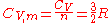whereis the heat capacity at constant volume of the gasis the molar heat capacity at constant volume of the gas
N is the total number of atoms present in the container
n is the number of moles
Mole (unit)
The mole is a unit of measurement used in chemistry to express amounts of a chemical substance, defined as an amount of a substance that contains as many elementary entities as there are atoms in 12 grams of pure carbon-12 , the isotope of carbon with atomic weight 12. This corresponds to a value...

of atoms present in the container (n is the ratio of N and Avogadro’s number
Avogadro's number
In chemistry and physics, the Avogadro constant is defined as the ratio of the number of constituent particles N in a sample to the amount of substance n through the relationship NA = N/n. Thus, it is the proportionality factor that relates the molar mass of an entity, i.e...

)
R is the ideal gas constant, (8.314570 J/(mol·K). R is equal to the product of Boltzmann’s constant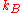and Avogadro’s number

The following table shows experimental molar constant volume heat capacity measurements taken for each noble monatomic gas (at 1 atm and 25 °C):
Monatomic gas CV, m (J/(mol·K)) CV, m/R
He 12.5 1.50
Ne 12.5 1.50
Ar 12.5 1.50
Kr 12.5 1.50
Xe 12.5 1.50

It is apparent from the table that the experimental heat capacities of the monatomic noble gases agrees with this simple application of statistical mechanics to a very high degree.

The molar heat capacity of a monatomic gas at constant pressure is then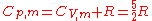### Diatomic gas

In the somewhat more complex case of an ideal gas of diatomic molecules, the presence of internal degrees of freedom are apparent. In addition to the three translational degrees of freedom, there are rotational and vibrational degrees of freedom. In general, the number of degrees of freedom, f, in a molecule with na atoms is 3na: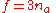Mathematically, there are a total of three rotational degrees of freedom, one corresponding to rotation about each of the axes of three dimensional space. However, in practice only the existence of two degrees of rotational freedom for linear molecules will be considered. This approximation is valid because the moment of inertia about the internuclear axis is vanishingly small with respect other moments of inertia in the molecule (this is due to the extremely small radii of the atomic nuclei, compared to the distance between them in a molecule). Quantum mechanically, it can be shown that the interval between successive rotational energy eigenstates is inversely proportional to the moment of inertia about that axis. Because the moment of inertia about the internuclear axis is vanishingly small relative to the other two rotational axes, the energy spacing can be considered so high that no excitations of the rotational state can possibly occur unless the temperature is extremely high. It is easy to calculate the expected number of vibrational degrees of freedom (or vibrational modes). There are three degrees of translational freedom, and two degrees of rotational freedom, therefore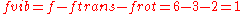Each rotational and translational degree of freedom will contribute R/2 in the total molar heat capacity of the gas. Each vibrational mode will contribute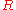to the total molar heat capacity, however. This is because for each vibrational mode, there is a potential and kinetic energy component. Both the potential and kinetic components will contribute R/2 to the total molar heat capacity of the gas. Therefore, a diatomic molecule would be expected to have a molar constant-volume heat capacity ofwhere the terms originate from the translational, rotational, and vibrational degrees of freedom, respectively.

The following is a table of some molar constant-volume heat capacities of various diatomic gases at standard temperature (25 oC = 298 K)
Diatomic gas CV, m (J/(mol·K)) CV, m / R
H2 20.18 2.427
CO 20.2 2.43
N2 19.9 2.39
Cl2 24.1 3.06
Br2 (vapour) 28.2 3.39

From the above table, clearly there is a problem with the above theory. All of the diatomics examined have heat capacities that are lower than those predicted by the equipartition theorem
Equipartition theorem
In classical statistical mechanics, the equipartition theorem is a general formula that relates the temperature of a system with its average energies. The equipartition theorem is also known as the law of equipartition, equipartition of energy, or simply equipartition...

, except Br2. However, as the atoms composing the molecules become heavier, the heat capacities move closer to their expected values. One of the reasons for this phenomenon is the quantization of vibrational, and to a lesser extent, rotational states. In fact, if it is assumed that the molecules remain in their lowest energy vibrational state because the inter-level energy spacings for vibration-energies are large, the predicted molar constant volume heat capacity for a diatomic molecule becomes just that from the contributions of translation and rotation: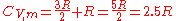which is a fairly close approximation of the heat capacities of the lighter molecules in the above table. If the quantum harmonic oscillator
Harmonic oscillator
In classical mechanics, a harmonic oscillator is a system that, when displaced from its equilibrium position, experiences a restoring force, F, proportional to the displacement, x: \vec F = -k \vec x \, where k is a positive constant....

approximation is made, it turns out that the quantum vibrational energy level spacings are actually inversely proportional to the square root of the reduced mass
Reduced mass
Reduced mass is the "effective" inertial mass appearing in the two-body problem of Newtonian mechanics. This is a quantity with the unit of mass, which allows the two-body problem to be solved as if it were a one-body problem. Note however that the mass determining the gravitational force is not...

of the atoms composing the diatomic molecule. Therefore, in the case of the heavier diatomic molecules such as chlorine or bromine, the quantum vibrational energy level spacings become finer, which allows more excitations into higher vibrational levels at lower temperatures. This limit for storing heat capacity in vibrational modes, as discussed above, becomes 7"R" /2 = 3.5 R per mole, which is fairly consistent with the measured value for Br2 at room temperature. As temperatures rise, all diatomic gases approach this value.

### General gas phase

The specific heat of the gas is best conceptualized in terms of the degrees of freedom
Degrees of freedom (physics and chemistry)
A degree of freedom is an independent physical parameter, often called a dimension, in the formal description of the state of a physical system...

of an individual molecule. The different degrees of freedom correspond to the different ways in which the molecule may store energy. The molecule may store energy in its translational motion according to the formula:where m  is the mass of the molecule andis velocity of the center of mass of the molecule. Each direction of motion constitutes a degree of freedom, so that there are three translational degrees of freedom.

In addition, a molecule may have rotational motion. The kinetic energy of rotational motion is generally expressed as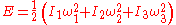where I  is the moment of inertia
Moment of inertia
In classical mechanics, moment of inertia, also called mass moment of inertia, rotational inertia, polar moment of inertia of mass, or the angular mass, is a measure of an object's resistance to changes to its rotation. It is the inertia of a rotating body with respect to its rotation...

tensor of the molecule, andis the angular velocity
Angular velocity
In physics, the angular velocity is a vector quantity which specifies the angular speed of an object and the axis about which the object is rotating. The SI unit of angular velocity is radians per second, although it may be measured in other units such as degrees per second, revolutions per...

pseudo-vector (in a coordinate system aligned with the principle axes of the molecule). In general, then, there will be three additional degrees of freedom corresponding to the rotational motion of the molecule, (For linear molecules one of the inertia tensor terms vanishes and there are only two rotational degrees of freedom). The degrees of freedom corresponding to translations and rotations are called the rigid degrees of freedom, since they do not involve any deformation of the molecule.

The motions of the atoms in a molecule which are not part of its gross translational motion or rotation may be classified as vibrational motions. It can be shown that if there are n atoms in the molecule, there will be as many as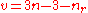vibrational degrees of freedom, whereis the number of rotational degrees of freedom. A vibrational degree of freedom corresponds to a specific way in which all the atoms of a molecule can vibrate. The actual number of possible vibrations may be less than this maximal one, due to various symmetries.

For example, triatomic nitrous oxide N2O will have only 2 degrees of rotational freedom (since it is a linear molecule) and contains n=3 atoms: thus the number of possible vibrational degrees of freedom will be v = (3*3)-3-2 = 4. There are four ways or "modes" in which the three atoms can vibrate, corresponding to 1) A mode in which an atom at each end of the molecule moves away from, or towards, the center atom at the same time, 2) a mode in which either end atom moves asynchronously with regard to the other two, and 3) and 4) two modes in which the molecule bends out of line, from the center, in the two possible planar directions that are orthogonal to its axis. Each vibrational degree of freedom confers TWO total degrees of freedom, since vibrational energy mode partitions into 1 kinetic and 1 potential mode. This would give nitrous oxide 3 translational, 2 rotational, and 4 vibrational modes (but these last giving 8 vibrational degrees of freedom), for storing energy. This is a total of f = 3+2+8 = 13 total energy-storing degrees of freedom, for N2O.

For a bent molecule like water H2O, a similar calculation gives 9-3-3 = 3 modes of vibration, and 3 (translational) + 3 (rotational) + 6(vibratonal) = 12 degrees of freedom.

### The storage of energy into degrees of freedom

If the molecule could be entirely described using classical mechanics, then the theorem of equipartition of energy could be used to predict that each degree of freedom would have an average energy in the amount of (1/2)kT  where k  is Boltzmann’s constant and T  is the temperature. Our calculation of the constant-volume heat capacity would be straightforward. Each molecule would be holding, on average, an energy of (f/2)kT  where f  is the total number of degrees of freedom in the molecule. Note that Nk = R if N is Avogadro's number
Avogadro's number
In chemistry and physics, the Avogadro constant is defined as the ratio of the number of constituent particles N in a sample to the amount of substance n through the relationship NA = N/n. Thus, it is the proportionality factor that relates the molar mass of an entity, i.e...

, which is the case in considering the heat capacity of a mole of molecules. Thus, the total internal energy of the gas would be (f/2)NkT  where N  is the total number of molecules. The heat capacity (at constant volume) would then be a constant (f/2)Nk  the mole-specific heat capacity would be (f/2)R  the molecule-specific heat capacity would be (f/2)k  and the dimensionless heat capacity would be just f/2. Here again, each vibrational degree of freedom contributes 2f. Thus, a mole of nitrous oxide would have a total constant-volume heat capacity (including vibration) of (13/2)R by this calculation.

In summary, the molar heat capacity (mole-specific heat capacity) of an ideal gas with f degrees of freedom is given by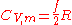This equation applies to all polyatomic gases, if the degrees of freedom are known.

The constant-pressure heat capacity for any gas would exceed this by an extra factor of R (see Mayer
Julius Robert von Mayer
Julius Robert von Mayer was a German physician and physicist and one of the founders of thermodynamics...

's relation, above). As example Cp would be a total of (15/2)R/mole for nitrous oxide.

### The effect of quantum energy levels in storing energy in degrees of freedom

The various degrees of freedom cannot generally be considered to obey classical mechanics, however. Classically, the energy residing in each degree of freedom is assumed to be continuous—it can take on any positive value, depending on the temperature. In reality, the amount of energy that may reside in a particular degree of freedom is quantized: It may only be increased and decreased in finite amounts. A good estimate of the size of this minimum amount is the energy of the first excited state of that degree of freedom above its ground state. For example, the first vibrational state of the hydrogen chloride (HCl) molecule has an energy of about 5.74 × 10−20 joule. If this amount of energy were deposited in a classical degree of freedom, it would correspond to a temperature of about 4156 K.

If the temperature of the substance is so low that the equipartition energy of (1/2)kT  is much smaller than this excitation energy, then there will be little or no energy in this degree of freedom. This degree of freedom is then said to be “frozen out". As mentioned above, the temperature corresponding to the first excited vibrational state of HCl is about 4156 K. For temperatures well below this value, the vibrational degrees of freedom of the HCl molecule will be frozen out. They will contain little energy and will not contribute to the thermal energy or the heat capacity of HCl gas.

### Energy storage mode "freeze-out" temperatures

It can be seen that for each degree of freedom there is a critical temperature at which the degree of freedom “unfreezes” and begins to accept energy in a classical way. In the case of translational degrees of freedom, this temperature is that temperature at which the thermal wavelength of the molecules is roughly equal to the size of the container. For a container of macroscopic size (e.g. 10 cm) this temperature is extremely small and has no significance, since the gas will certainly liquify or freeze before this low temperature is reached. For any real gas translational degrees of freedom may be considered to always be classical and contain an average energy of (3/2)kT  per molecule.

The rotational degrees of freedom are the next to “unfreeze". In a diatomic gas, for example, the critical temperature for this transition is usually a few tens of kelvins, although with a very light molecule such as hydrogen the rotational energy levels will be spaced so widely that rotational heat capacity may not completely "unfreeze" until considerably higher temperatures are reached. Finally, the vibrational degrees of freedom are generally the last to unfreeze. As an example, for diatomic gases, the critical temperature for the vibrational motion is usually a few thousands of kelvins, and thus for the nitrogen in our example at room temperature, no vibration modes would be exited, and the constant-volume heat capacity at room temperature is (5/2)R/mole, not (7/2)R/mole. As seen above, with some unusually heavy gases such as iodine gas I2, or bromine gas Br2, some vibrational heat capacity may be observed even at room temperatures.

It should be noted that it has been assumed that atoms have no rotational or internal degrees of freedom. This is in fact untrue. For example, atomic electrons can exist in excited states and even the atomic nucleus can have excited states as well. Each of these internal degrees of freedom are assumed to be frozen out due to their relatively high excitation energy. Nevertheless, for sufficiently high temperatures, these degrees of freedom cannot be ignored. In a few exceptional cases, such molecular electronic transitions are of sufficiently low energy that they contribute to heat capacity at room temperature, or even at cryogenic temperatures. One example of an electronic transition degree of freedom which contributes heat capacity at standard temperature is that of nitric oxide (NO), in which the single electron in an anti-bonding molecular orbital has energy transitions which contribute to the heat capacity of the gas even at room temperature.

An example of a nuclear magnetic transition degree of freedom which is of importance to heat capacity, is the transition which converts the spin isomers of hydrogen
Spin isomers of hydrogen
Molecular hydrogen occurs in two isomeric forms, one with its two proton spins aligned parallel , the other with its two proton spins aligned antiparallel...

gas (H2) into each other. At room temperature, the proton spins of hydrogen gas are aligned 75% of the time, resulting in orthohydrogen when they are. Thus, some thermal energy has been stored in the degree of freedom available when parahydrogen (in which spins are anti-aligned) absorbs energy, and is converted to the higher energy ortho form. However, at the temperature of liquid hydrogen, not enough heat energy is available to produce orthohydrogen (that is, the transition energy between forms is large enough to "freeze out" at this low temperature), and thus the parahydrogen form predominates. The heat capacity of the transition is sufficient to release enough heat, as orthohydrogen converts to the lower-energy parahydrogen, to boil the hydrogen liquid to gas again, if this evolved heat is not removed with a catalyst after the gas has been cooled and condensed. This example also illustrates the fact that some modes of storage of heat may not be in constant equilibrium with each other in substances, and heat absorbed or released from such phase changes may "catch up" with temperature changes of substances, only after a certain time. In other words, the heat evolved and absorbed from the ortho-para isomeric transition contributes to the heat capacity of hydrogen on long time-scales, but not on short time-scales. These time scales may also depend on the presence of a catalyst.

Less exotic phase-changes may contribute to the heat-capacity of substances and systems, as well, as (for example) when water is converted back and forth from solid to liquid or gas form. Phase changes store heat energy entirely in breaking the bonds of the potential energy interactions between molecules of a substance. As in the case of hydrogen, it is also possible for phase changes to be hindered as the temperature drops, so that they do not catch up and become apparent, without a catalyst. For example, it is possible to supercool liquid water to below the freezing point, and not observe the heat evolved when the water changes to ice, so long as the water remains liquid. This heat appears instantly when the water freezes.

### Solid phase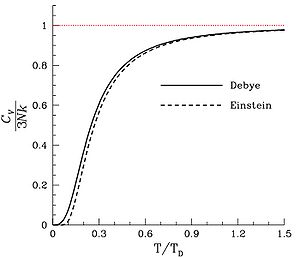For matter in a crystalline solid phase, the Dulong-Petit law, which was discovered empirically, states that the mole-specific heat capacity assumes the value 3 R. Indeed, for solid metallic chemical elements at room temperature, molar heat capacities range from about 2.8 R to 3.4 R. Large exceptions involve solids composed of light, tightly bonded atoms such as beryllium
Beryllium
Beryllium is the chemical element with the symbol Be and atomic number 4. It is a divalent element which occurs naturally only in combination with other elements in minerals. Notable gemstones which contain beryllium include beryl and chrysoberyl...

at 2.0 R, and diamond
Diamond
In mineralogy, diamond is an allotrope of carbon, where the carbon atoms are arranged in a variation of the face-centered cubic crystal structure called a diamond lattice. Diamond is less stable than graphite, but the conversion rate from diamond to graphite is negligible at ambient conditions...

at only 0.735 R. The latter conditions create large quantum vibrational energy spacing, so that many vibrational modes are not available (are frozen out) at room temperature.

The theoretical maximum heat capacity for larger and larger multi-atomic gases at higher temperatures also approaches the Dulong-Petit limit of 3 R, so long as this is calculated per mole of atoms, not molecules. The reason is that, in theory, gases with very large molecules have almost the same high-temperature heat capacity as solids, lacking only the (small) heat capacity contribution that comes from potential energy that cannot be stored between separate molecules in a gas.

The Dulong-Petit limit results from the equipartition theorem
Equipartition theorem
In classical statistical mechanics, the equipartition theorem is a general formula that relates the temperature of a system with its average energies. The equipartition theorem is also known as the law of equipartition, equipartition of energy, or simply equipartition...

, and as such is only valid in the classical limit of a microstate continuum
Microstate continuum
A microstate continuum is the fluctuation spectrum of a thermodynamic system in the classical limit of high temperatures. Classical here is to be understood in opposition to quantum statistical mechanics.-See also:...

, which is a high temperature limit. For light and non-metallic elements, as well as most of the common molecular solids based on carbon compounds at standard ambient temperature, quantum effects may also play an important role, as they do in multi-atomic gases. These effects usually combine to give heat capacities lower than 3 R per mole of atoms in the solid, although in molecular solids, heat capacities calculated per mole of molecules in molecular solids may be more than 3 R. For example, the heat capacity of water ice at the melting point is about 4.6 R per mole of molecules, but only 1.5 R per mole of atoms. The lower than 3 R number "per atom" (as is the case with diamond and beryllium) results from the “freezing out” of possible vibration modes for light atoms at suitably low temperatures, just as in many low-mass-atom gases at room temperatures. Because of high crystal binding energies, these effects are seen in solids more often than liquids: for example the heat capacity of liquid water is twice that of ice at near the same temperature, and is again close to the 3 R per mole of atoms of the Dulong-Petit theoretical maximum.

For a more modern and precise analysis of the heat capacities of solids, especially at low temperatures, it is useful to use the idea of phonons. See Debye model
Debye model
In thermodynamics and solid state physics, the Debye model is a method developed by Peter Debye in 1912 for estimating the phonon contribution to the specific heat in a solid. It treats the vibrations of the atomic lattice as phonons in a box, in contrast to the Einstein model, which treats the...

.

The specific heat of amorphous materials has characteristic discontinuities at the glass transition temperature due to rearrangements that occur in the distribution of atoms. These discontinuities are frequently used to detect the glass transition temperature where a supercooled liquid transforms to a glass.

## Table of specific heat capacities

Note that especially high values, as for paraffin, water and ammonia, result from calculating specific heats in terms of moles of molecules. If specific heat is expressed per mole of atoms for these substances, few constant-volume values exceed the theoretical Dulong-Petit limit
Dulong–Petit law
The Dulong–Petit law, a chemical law proposed in 1819 by French physicists Pierre Louis Dulong and Alexis Thérèse Petit, states the classical expression for the molar specific heat capacity of a crystal...

of 25 J/(mol·K) = 3 R per mole of atoms.
Table of specific heat capacities at 25 °C (298.15 K) unless otherwise noted Notable minima and maxima are shown in maroon
Substance Phase
Phase (matter)
In the physical sciences, a phase is a region of space , throughout which all physical properties of a material are essentially uniform. Examples of physical properties include density, index of refraction, and chemical composition...

cp
J·g−1·K−1
Cp,m
J·mol−1·K−1
Cv,m
J·mol−1·K−1
Volumetric
heat capacity
Volumetric heat capacity
Volumetric heat capacity , also termed volume-specific heat capacity, describes the ability of a given volume of a substance to store internal energy while undergoing a given temperature change, but without undergoing a phase change...

J·cm−3·K−1
Air
Earth's atmosphere
The atmosphere of Earth is a layer of gases surrounding the planet Earth that is retained by Earth's gravity. The atmosphere protects life on Earth by absorbing ultraviolet solar radiation, warming the surface through heat retention , and reducing temperature extremes between day and night...

(Sea level, dry, 0 °C (273.15 K))
gas 1.0035 29.07 20.7643 0.001297
Air (typical room conditionsA) gas 1.012 29.19 20.85 0.00121
Aluminium
Aluminium
Aluminium or aluminum is a silvery white member of the boron group of chemical elements. It has the symbol Al, and its atomic number is 13. It is not soluble in water under normal circumstances....

solid 0.897 24.2 2.422
Ammonia
Ammonia
Ammonia is a compound of nitrogen and hydrogen with the formula . It is a colourless gas with a characteristic pungent odour. Ammonia contributes significantly to the nutritional needs of terrestrial organisms by serving as a precursor to food and fertilizers. Ammonia, either directly or...

liquid 4.700 80.08 3.263
Animal (incl. human) tissue  mixed 3.5 3.7*
Antimony
Antimony
Antimony is a toxic chemical element with the symbol Sb and an atomic number of 51. A lustrous grey metalloid, it is found in nature mainly as the sulfide mineral stibnite...

solid 0.207 25.2 1.386
Argon
Argon
Argon is a chemical element represented by the symbol Ar. Argon has atomic number 18 and is the third element in group 18 of the periodic table . Argon is the third most common gas in the Earth's atmosphere, at 0.93%, making it more common than carbon dioxide...

gas 0.5203 20.7862 12.4717
Arsenic
Arsenic
Arsenic is a chemical element with the symbol As, atomic number 33 and relative atomic mass 74.92. Arsenic occurs in many minerals, usually in conjunction with sulfur and metals, and also as a pure elemental crystal. It was first documented by Albertus Magnus in 1250.Arsenic is a metalloid...

solid 0.328 24.6 1.878
Beryllium
Beryllium
Beryllium is the chemical element with the symbol Be and atomic number 4. It is a divalent element which occurs naturally only in combination with other elements in minerals. Notable gemstones which contain beryllium include beryl and chrysoberyl...

solid 1.82 16.4 3.367
Bismuth
Bismuth
Bismuth is a chemical element with symbol Bi and atomic number 83. Bismuth, a trivalent poor metal, chemically resembles arsenic and antimony. Elemental bismuth may occur naturally uncombined, although its sulfide and oxide form important commercial ores. The free element is 86% as dense as lead...

solid 0.123 25.7 1.20
Cadmium
Cadmium
Cadmium is a chemical element with the symbol Cd and atomic number 48. This soft, bluish-white metal is chemically similar to the two other stable metals in group 12, zinc and mercury. Similar to zinc, it prefers oxidation state +2 in most of its compounds and similar to mercury it shows a low...

solid 0.231
Carbon dioxide
Carbon dioxide
Carbon dioxide is a naturally occurring chemical compound composed of two oxygen atoms covalently bonded to a single carbon atom...

CO2
gas 0.839* 36.94 28.46
Chromium
Chromium
Chromium is a chemical element which has the symbol Cr and atomic number 24. It is the first element in Group 6. It is a steely-gray, lustrous, hard metal that takes a high polish and has a high melting point. It is also odorless, tasteless, and malleable...

solid 0.449
Copper
Copper
Copper is a chemical element with the symbol Cu and atomic number 29. It is a ductile metal with very high thermal and electrical conductivity. Pure copper is soft and malleable; an exposed surface has a reddish-orange tarnish...

solid 0.385 24.47 3.45
Diamond
Diamond
In mineralogy, diamond is an allotrope of carbon, where the carbon atoms are arranged in a variation of the face-centered cubic crystal structure called a diamond lattice. Diamond is less stable than graphite, but the conversion rate from diamond to graphite is negligible at ambient conditions...

solid 0.5091 6.115 1.782
Ethanol
Ethanol
Ethanol, also called ethyl alcohol, pure alcohol, grain alcohol, or drinking alcohol, is a volatile, flammable, colorless liquid. It is a psychoactive drug and one of the oldest recreational drugs. Best known as the type of alcohol found in alcoholic beverages, it is also used in thermometers, as a...

liquid 2.44 112 1.925
Gasoline
Gasoline
Gasoline , or petrol , is a toxic, translucent, petroleum-derived liquid that is primarily used as a fuel in internal combustion engines. It consists mostly of organic compounds obtained by the fractional distillation of petroleum, enhanced with a variety of additives. Some gasolines also contain...

liquid 2.22 228 1.64
Glass
Glass
Glass is an amorphous solid material. Glasses are typically brittle and optically transparent.The most familiar type of glass, used for centuries in windows and drinking vessels, is soda-lime glass, composed of about 75% silica plus Na2O, CaO, and several minor additives...

solid 0.84
Gold
Gold
Gold is a chemical element with the symbol Au and an atomic number of 79. Gold is a dense, soft, shiny, malleable and ductile metal. Pure gold has a bright yellow color and luster traditionally considered attractive, which it maintains without oxidizing in air or water. Chemically, gold is a...

solid 0.129 25.42 2.492
Granite
Granite
Granite is a common and widely occurring type of intrusive, felsic, igneous rock. Granite usually has a medium- to coarse-grained texture. Occasionally some individual crystals are larger than the groundmass, in which case the texture is known as porphyritic. A granitic rock with a porphyritic...

solid 0.790 2.17
Graphite
Graphite
The mineral graphite is one of the allotropes of carbon. It was named by Abraham Gottlob Werner in 1789 from the Ancient Greek γράφω , "to draw/write", for its use in pencils, where it is commonly called lead . Unlike diamond , graphite is an electrical conductor, a semimetal...

solid 0.710 8.53 1.534
Helium
Helium
Helium is the chemical element with atomic number 2 and an atomic weight of 4.002602, which is represented by the symbol He. It is a colorless, odorless, tasteless, non-toxic, inert, monatomic gas that heads the noble gas group in the periodic table...

gas 5.1932 20.7862 12.4717
Hydrogen
Hydrogen
Hydrogen is the chemical element with atomic number 1. It is represented by the symbol H. With an average atomic weight of , hydrogen is the lightest and most abundant chemical element, constituting roughly 75% of the Universe's chemical elemental mass. Stars in the main sequence are mainly...

gas 14.30 28.82
Hydrogen sulfide
Hydrogen sulfide
Hydrogen sulfide is the chemical compound with the formula . It is a colorless, very poisonous, flammable gas with the characteristic foul odor of expired eggs perceptible at concentrations as low as 0.00047 parts per million...

H2S
gas 1.015* 34.60
Iron
Iron
Iron is a chemical element with the symbol Fe and atomic number 26. It is a metal in the first transition series. It is the most common element forming the planet Earth as a whole, forming much of Earth's outer and inner core. It is the fourth most common element in the Earth's crust...

solid 0.450 25.1 3.537
Lead
Lead
Lead is a main-group element in the carbon group with the symbol Pb and atomic number 82. Lead is a soft, malleable poor metal. It is also counted as one of the heavy metals. Metallic lead has a bluish-white color after being freshly cut, but it soon tarnishes to a dull grayish color when exposed...

solid 0.129 26.4 1.44
Lithium
Lithium
Lithium is a soft, silver-white metal that belongs to the alkali metal group of chemical elements. It is represented by the symbol Li, and it has the atomic number 3. Under standard conditions it is the lightest metal and the least dense solid element. Like all alkali metals, lithium is highly...

solid 3.58 24.8 1.912
Lithium
Lithium
Lithium is a soft, silver-white metal that belongs to the alkali metal group of chemical elements. It is represented by the symbol Li, and it has the atomic number 3. Under standard conditions it is the lightest metal and the least dense solid element. Like all alkali metals, lithium is highly...

at 181 °C
liquid 4.379 30.33 2.242
Magnesium
Magnesium
Magnesium is a chemical element with the symbol Mg, atomic number 12, and common oxidation number +2. It is an alkaline earth metal and the eighth most abundant element in the Earth's crust and ninth in the known universe as a whole...

solid 1.02 24.9 1.773
Mercury
Mercury (element)
Mercury is a chemical element with the symbol Hg and atomic number 80. It is also known as quicksilver or hydrargyrum...

liquid 0.1395 27.98 1.888
Methane
Methane
Methane is a chemical compound with the chemical formula . It is the simplest alkane, the principal component of natural gas, and probably the most abundant organic compound on earth. The relative abundance of methane makes it an attractive fuel...

at 2 °C
gas 2.191
Methanol
Methanol
Methanol, also known as methyl alcohol, wood alcohol, wood naphtha or wood spirits, is a chemical with the formula CH3OH . It is the simplest alcohol, and is a light, volatile, colorless, flammable liquid with a distinctive odor very similar to, but slightly sweeter than, ethanol...

liquid 2.597
Nitrogen
Nitrogen
Nitrogen is a chemical element that has the symbol N, atomic number of 7 and atomic mass 14.00674 u. Elemental nitrogen is a colorless, odorless, tasteless, and mostly inert diatomic gas at standard conditions, constituting 78.08% by volume of Earth's atmosphere...

gas 1.040 29.12 20.8
Neon
Neon
Neon is the chemical element that has the symbol Ne and an atomic number of 10. Although a very common element in the universe, it is rare on Earth. A colorless, inert noble gas under standard conditions, neon gives a distinct reddish-orange glow when used in either low-voltage neon glow lamps or...

gas 1.0301 20.7862 12.4717
Oxygen
Oxygen
Oxygen is the element with atomic number 8 and represented by the symbol O. Its name derives from the Greek roots ὀξύς and -γενής , because at the time of naming, it was mistakenly thought that all acids required oxygen in their composition...

gas 0.918 29.38
Paraffin wax
Paraffin
In chemistry, paraffin is a term that can be used synonymously with "alkane", indicating hydrocarbons with the general formula CnH2n+2. Paraffin wax refers to a mixture of alkanes that falls within the 20 ≤ n ≤ 40 range; they are found in the solid state at room temperature and begin to enter the...

solid 2.5 900 2.325
Polyethylene
Polyethylene
Polyethylene or polythene is the most widely used plastic, with an annual production of approximately 80 million metric tons...

(rotomolding grade)
solid 2.3027
Polyethylene
Polyethylene
Polyethylene or polythene is the most widely used plastic, with an annual production of approximately 80 million metric tons...

(rotomolding grade)
liquid 2.9308
Silica
Silicon dioxide
The chemical compound silicon dioxide, also known as silica , is an oxide of silicon with the chemical formula '. It has been known for its hardness since antiquity...

(fused)
solid 0.703 42.2 1.547
Silver
Silver
Silver is a metallic chemical element with the chemical symbol Ag and atomic number 47. A soft, white, lustrous transition metal, it has the highest electrical conductivity of any element and the highest thermal conductivity of any metal...

solid 0.233 24.9 2.44
Sodium
Sodium
Sodium is a chemical element with the symbol Na and atomic number 11. It is a soft, silvery-white, highly reactive metal and is a member of the alkali metals; its only stable isotope is 23Na. It is an abundant element that exists in numerous minerals, most commonly as sodium chloride...

solid 1.230
Tin
Tin
Tin is a chemical element with the symbol Sn and atomic number 50. It is a main group metal in group 14 of the periodic table. Tin shows chemical similarity to both neighboring group 14 elements, germanium and lead and has two possible oxidation states, +2 and the slightly more stable +4...

solid 0.227
Titanium
Titanium
Titanium is a chemical element with the symbol Ti and atomic number 22. It has a low density and is a strong, lustrous, corrosion-resistant transition metal with a silver color....

solid 0.523
Tungsten
Tungsten
Tungsten , also known as wolfram , is a chemical element with the chemical symbol W and atomic number 74.A hard, rare metal under standard conditions when uncombined, tungsten is found naturally on Earth only in chemical compounds. It was identified as a new element in 1781, and first isolated as...

solid 0.134 24.8 2.58
Uranium
Uranium
Uranium is a silvery-white metallic chemical element in the actinide series of the periodic table, with atomic number 92. It is assigned the chemical symbol U. A uranium atom has 92 protons and 92 electrons, of which 6 are valence electrons...

solid 0.116 27.7 2.216
Water at 100 °C (steam) gas 2.080 37.47 28.03
Water at 25 °C liquid 4.1813 75.327 74.53 4.1796
Water at 100 °C liquid 4.1813 75.327 74.53 4.2160
Water at −10 °C (ice) solid 2.11 38.09 1.938
Zinc
Zinc
Zinc , or spelter , is a metallic chemical element; it has the symbol Zn and atomic number 30. It is the first element in group 12 of the periodic table. Zinc is, in some respects, chemically similar to magnesium, because its ion is of similar size and its only common oxidation state is +2...

solid 0.387 25.2 2.76
Substance Phase
Phase (matter)
In the physical sciences, a phase is a region of space , throughout which all physical properties of a material are essentially uniform. Examples of physical properties include density, index of refraction, and chemical composition...

Cp
J/(g·K)
Cp,m
J/(mol·K)
Cv,m
J/(mol·K)
Volumetric
heat capacity
Volumetric heat capacity
Volumetric heat capacity , also termed volume-specific heat capacity, describes the ability of a given volume of a substance to store internal energy while undergoing a given temperature change, but without undergoing a phase change...

J/(cm3·K)

A Assuming an altitude of 194 metres above mean sea level (the world–wide median altitude of human habitation), an indoor temperature of 23 °C, a dewpoint of 9 °C (40.85% relative humidity), and 760 mm–Hg sea level–corrected barometric pressure (molar water vapor content = 1.16%).

*Derived data by calculation. This is for water-rich tissues such as brain. The whole-body average figure for mammals is approximately 2.9 J/(cm3·K)

## Specific heat capacity of building materials

(Usually of interest to builders and solar designers)
Specific heat capacity of building materials
Substance Phase cp
J/(g·K)
Asphalt
Asphalt
Asphalt or , also known as bitumen, is a sticky, black and highly viscous liquid or semi-solid that is present in most crude petroleums and in some natural deposits, it is a substance classed as a pitch...

solid 0.920
Brick
Brick
A brick is a block of ceramic material used in masonry construction, usually laid using various kinds of mortar. It has been regarded as one of the longest lasting and strongest building materials used throughout history.-History:...

solid 0.840
Concrete
Concrete
Concrete is a composite construction material, composed of cement and other cementitious materials such as fly ash and slag cement, aggregate , water and chemical admixtures.The word concrete comes from the Latin word...

solid 0.880
Glass
Glass
Glass is an amorphous solid material. Glasses are typically brittle and optically transparent.The most familiar type of glass, used for centuries in windows and drinking vessels, is soda-lime glass, composed of about 75% silica plus Na2O, CaO, and several minor additives...

, silica
solid 0.840
Glass
Glass
Glass is an amorphous solid material. Glasses are typically brittle and optically transparent.The most familiar type of glass, used for centuries in windows and drinking vessels, is soda-lime glass, composed of about 75% silica plus Na2O, CaO, and several minor additives...

, crown
solid 0.670
Glass
Glass
Glass is an amorphous solid material. Glasses are typically brittle and optically transparent.The most familiar type of glass, used for centuries in windows and drinking vessels, is soda-lime glass, composed of about 75% silica plus Na2O, CaO, and several minor additives...

, flint
solid 0.503
Glass
Glass
Glass is an amorphous solid material. Glasses are typically brittle and optically transparent.The most familiar type of glass, used for centuries in windows and drinking vessels, is soda-lime glass, composed of about 75% silica plus Na2O, CaO, and several minor additives...

, pyrex
solid 0.753
Granite
Granite
Granite is a common and widely occurring type of intrusive, felsic, igneous rock. Granite usually has a medium- to coarse-grained texture. Occasionally some individual crystals are larger than the groundmass, in which case the texture is known as porphyritic. A granitic rock with a porphyritic...

solid 0.790
Gypsum
Gypsum
Gypsum is a very soft sulfate mineral composed of calcium sulfate dihydrate, with the chemical formula CaSO4·2H2O. It is found in alabaster, a decorative stone used in Ancient Egypt. It is the second softest mineral on the Mohs Hardness Scale...

solid 1.090
Marble
Marble
Marble is a metamorphic rock composed of recrystallized carbonate minerals, most commonly calcite or dolomite.Geologists use the term "marble" to refer to metamorphosed limestone; however stonemasons use the term more broadly to encompass unmetamorphosed limestone.Marble is commonly used for...

, mica
Mica
The mica group of sheet silicate minerals includes several closely related materials having highly perfect basal cleavage. All are monoclinic, with a tendency towards pseudohexagonal crystals, and are similar in chemical composition...

solid 0.880
Sand
Sand
Sand is a naturally occurring granular material composed of finely divided rock and mineral particles.The composition of sand is highly variable, depending on the local rock sources and conditions, but the most common constituent of sand in inland continental settings and non-tropical coastal...

solid 0.835
Soil
Soil
Soil is a natural body consisting of layers of mineral constituents of variable thicknesses, which differ from the parent materials in their morphological, physical, chemical, and mineralogical characteristics...

solid 0.800
Wood
Wood
Wood is a hard, fibrous tissue found in many trees. It has been used for hundreds of thousands of years for both fuel and as a construction material. It is an organic material, a natural composite of cellulose fibers embedded in a matrix of lignin which resists compression...

solid 1.7 (1.2 to 2.3)
Substance Phase cp
J/(g·K)

## See also

• Quantum statistical mechanics
Quantum statistical mechanics
Quantum statistical mechanics is the study of statistical ensembles of quantum mechanical systems. A statistical ensemble is described by a density operator S, which is a non-negative, self-adjoint, trace-class operator of trace 1 on the Hilbert space H describing the quantum system. This can be...

• Heat capacity ratio
Heat capacity ratio
The heat capacity ratio or adiabatic index or ratio of specific heats, is the ratio of the heat capacity at constant pressure to heat capacity at constant volume . It is sometimes also known as the isentropic expansion factor and is denoted by \gamma or \kappa . The latter symbol kappa is...

• Statistical mechanics
Statistical mechanics
Statistical mechanics or statistical thermodynamicsThe terms statistical mechanics and statistical thermodynamics are used interchangeably...

• Thermodynamic equations
Thermodynamic equations
Thermodynamics is expressed by a mathematical framework of thermodynamic equations which relate various thermodynamic quantities and physical properties measured in a laboratory or production process...

• Thermodynamic databases for pure substances
Thermodynamic databases for pure substances
Thermodynamic databases contain information about thermodynamic properties for substances, the most important being enthalpy, entropy, and Gibbs free energy. Numerical values of these thermodynamic properties are collected as tables or are calculated from thermodynamic datafiles...

• Heat equation
Heat equation
The heat equation is an important partial differential equation which describes the distribution of heat in a given region over time...

• Heat transfer coefficient
• Latent heat
Latent heat
Latent heat is the heat released or absorbed by a chemical substance or a thermodynamic system during a process that occurs without a change in temperature. A typical example is a change of state of matter, meaning a phase transition such as the melting of ice or the boiling of water. The term was...

• Material properties (thermodynamics)
Material properties (thermodynamics)
The thermodynamic properties of materials are intensive thermodynamic parameters which are specific to a given material. Each is directly related to a second order differential of a thermodynamic potential...

• Joback method
Joback method
The Joback method predicts eleven important and commonly used pure component thermodynamic properties from molecular structure only.- Group Contribution Method :The Joback method is a group contribution method...

(Estimation of heat capacities)
• Specific melting heat
• Specific heat of vaporization
• Volumetric heat capacity
Volumetric heat capacity
Volumetric heat capacity , also termed volume-specific heat capacity, describes the ability of a given volume of a substance to store internal energy while undergoing a given temperature change, but without undergoing a phase change...

• Thermal mass
Thermal mass
Thermal mass is a concept in building design which describes how the mass of the building provides "inertia" against temperature fluctuations, sometimes known as the thermal flywheel effect...

• R-value (insulation)
R-value (insulation)
The R-value is a measure of thermal resistance used in the building and construction industry. Under uniform conditions it is the ratio of the temperature difference across an insulator and the heat flux through it or R = \Delta T/\dot Q_A.The R-value being discussed is the unit thermal resistance...

The source of this article is wikipedia, the free encyclopedia.  The text of this article is licensed under the GFDL.